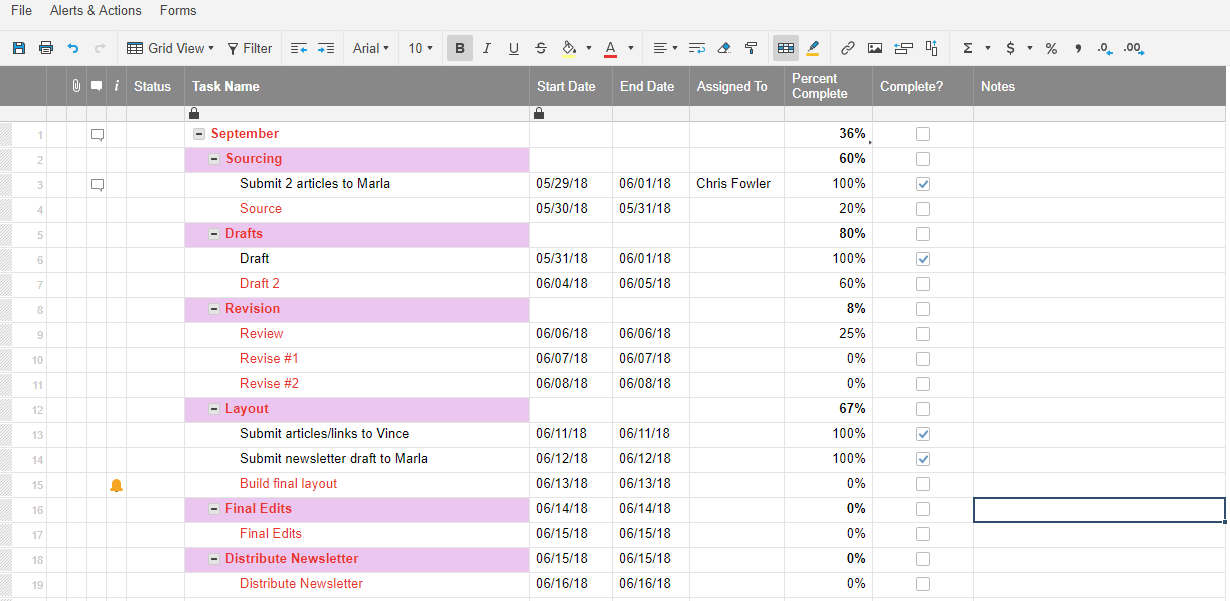# Nested IF Statement Formula

Hello Team,

This would be a great formula to incorporate into my daily SmartSheets process within my organization. I would like to know how to create a nested IF statement if this is the correct formula to use, that uses an RYG (red, yellow, green) light flag formula to set the status of a task based on its percent complete. I have not been able to figure this one out. If a task is less than 50% complete, turn the RYG light "Red" If a task is between 50-99% complete, turn the RYG light "Yellow" If a task is 100% complete, turn the RYG light "Green"

Thank you,

• Try this formula. Replace "Percentage complete column name" with your own column name. And replace 23 througout the formula with whatever row you are referencing.

=IF([Percentage complete column name]23 < .5, "Red", IF(AND([Percentage complete column name]23 >= .5, [Percentage complete column name]23 < 1), IF([Percentage complete column name]23 = 1, "Green")))

• Mike, I also had the same question and tried your formula with no luck.  I changed the % Completed to reference the appropriate cell but kept getting an "Unparsable" error.

• Hello Mike,

What row and column should the formula be inserted to?

Thanks Mike• Whoops I forgot the then response for the yellow section. Try this rewrite. Make sure to update the column name and the row number.

=IF([Percentage complete column name]23 < .5, "Red", IF(AND([Percentage complete column name]23 >= .5, [Percentage complete column name]23 < 1), "Yellow", IF([Percentage complete column name]23 = 1, "Green")))

• Hello Mike,

I cant get it to work, also which row and column should the formula be added.

Thanks,

• Hello Mike,

Got it and it works!

Thank you Sir,

• You are welcome! Glad I could be of help!

• That worked for me as well.  Thanks for the help and quick response.

• I'm amazed that this is not built into Smartsheet. If it's not included in the Project Settings as a configuration item, at least create a template for these poor users. Seriously.... what other question is asked more on these Community forums other than "Automate RYG"?

• I agree. The issue of percentages displaying as decimals is very hidden and not intuitive. If not for greats like J. Craig Willams I would not have know this information.

• Was able to tailor this to my sheet as well - thank you!

=IF([% Complete]23 < .5, "Red", IF(AND([% Complete]23 >= .5, [% Complete]23 < 1), "Yellow", IF([% Complete]23 = 1, "Green")))

Please note that the #s next to [% Complete] reflect the row #.

## Help Article Resources

Want to practice working with formulas directly in Smartsheet?

Check out the Formula Handbook template!